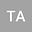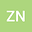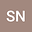A fuzzy method for solving fuzzy fractional differential equations based on the generalized fuzzy Taylor expansion
•••• +2Zahra Noeiaghdam
Shahed University
Author ProfileSouth Ural State University (National Research University)
Author ProfileSoheil Salahshour
Bahcesehir University
Author ProfileJuan Nieto
University of Santiago de Compostela
Author Profile## Abstract

In many mathematical types of research, in order to solve the fuzzy fractional differential equations, we should transform these problems into crisp corresponding problems and by solving them the approximate solution can be obtained. The aim of this paper is to present a new direct method to solve the fuzzy fractional differential equations without this transformation. In this work, the fuzzy generalized Taylor expansion by using the sense of fuzzy Caputo fractional derivative for fuzzy-valued functions is presented. For solving fuzzy fractional differential equations, the fuzzy generalized Euler's method is applied. In order to show the accuracy and efficiency of the presented method, the local and global truncation errors are determined. Moreover, the consistency, the convergence and the stability of the generalized Euler's method are proved in detail. Eventually, the numerical examples, especially in the switching point case, show the flexibility and the capability of the presented method.